# Generating Reflectance Curves from sRGB Triplets (Page 5)

#### Least Log Slope Squared (LLSS) Method

It is generally not a good idea to allow reflectance curves with negative values. Not only is this physically meaningless, but it also can cause problems down the road when the reflectance curves are used in other computations. For example, when modeling subtractive color mixture, it may be necessary to require the reflectance curves to be strictly positive.

One way to modify the LSS method to keep reflectances positive is to operate the algorithm in the space of the logarithm of reflectance values,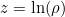. I call this the Least Log Slope Squared (LLSS) method: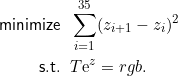This new optimization is not as easy to solve as the previous one. Nevertheless, the Lagrangian formulation can still be used, giving rise to a system of 39 nonlinear equations and 39 unknowns:where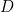is the same 36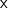36 tridiagonal matrix presented earlier.

Newton’s method solves this system of equations with ease, typically in just a few iterations. Forming the Jacobian matrix,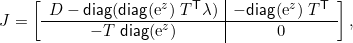the change in the variables with each Newton iteration is found by solving the linear system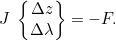Here is a Matlab program for the LLSS (Least Log Slope Squared) method. It also works in the free Octave software. I added a check for the special case of sRGB = (0,0,0), which simply returns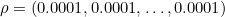. This is necessary since the log formulation is not able to create a reflectance of exactly zero (nor is that desirable in some applications). It can come very close to zero as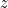approaches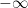, but it is numerically better to handle this one case specially. I chose the value of 0.0001 because it is the largest power of ten that translates back to an integer sRGB triplet of (0,0,0).

If you prefer not to work in a programming environment, here is an Excel spreadsheet that implements the LLSS method. Just follow the instructions at the top of the spreadsheet. It uses the “Solver” add-in within Excel, which you may need to load. Here are instructions for doing that.

The LLSS method requires substantially more computational effort than the previous two methods. Each iteration of Newton’s method requires the solution of 39 linear equations in 39 unknowns.

This Matlab program has also been tested in Octave and was found to work fine.